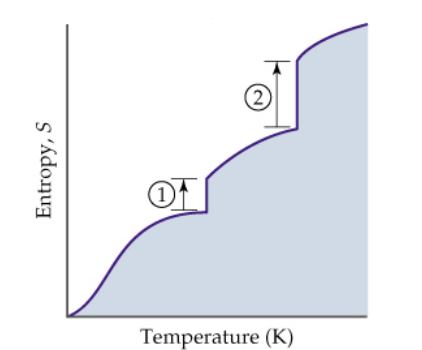Chemistry Practice Problems Entropy Practice Problems Solution: The diagram below shows the variation in entropy w...

# Solution: The diagram below shows the variation in entropy with temperature for a substance that is a gas at the highest temperature depicted. Accordingly, which of the following statements is not true? (a) The “zero point” is explained by the Second Law of Thermodynamics. (b) The entropy of a substance increases with temperature. (c) A solid has the least dispersed energy and the lowest entropy. (d) The vertical line 1 corresponds to ΔSfusion. (e) The increase in entropy for liquid  ⇌ gas is greater than for solid ⇌ liquid

###### Problem

The diagram below shows the variation in entropy with temperature for a substance that is a gas at the highest temperature depicted. Accordingly, which of the following statements is not true?

(a) The “zero point” is explained by the Second Law of Thermodynamics.

(b) The entropy of a substance increases with temperature.

(c) A solid has the least dispersed energy and the lowest entropy.

(d) The vertical line 1 corresponds to ΔSfusion.

(e) The increase in entropy for liquid  ⇌ gas is greater than for solid ⇌ liquidEntropy

Entropy

#### Q. Rank the standard molar entropy of the following from lowest to highest. 1. H2O2 (l) 2. H2O (g) 3. H2O (l) 4. H2O2 (aq) a) 4 &lt; 1 &lt; 3 &lt; 2...

Solved • Tue May 17 2016 11:56:21 GMT-0400 (EDT)

Entropy

#### Q. Consider the following processes (at 298 K) given below and determine for which is ΔS positive?

Solved • Tue May 17 2016 11:20:18 GMT-0400 (EDT)

Entropy

#### Q. For which reaction, carried out at standard conditions, would both the enthalpy and entropy changes drive the reaction in the same direction? a) 2 H2...

Solved • Mon May 09 2016 17:47:49 GMT-0400 (EDT)

Entropy

#### Q. In which process is entropy decreased ? a) dissolving sugar in water b) expanding a gas c) evaporating a liquid d) freezing water

Solved • Mon May 09 2016 16:55:48 GMT-0400 (EDT)